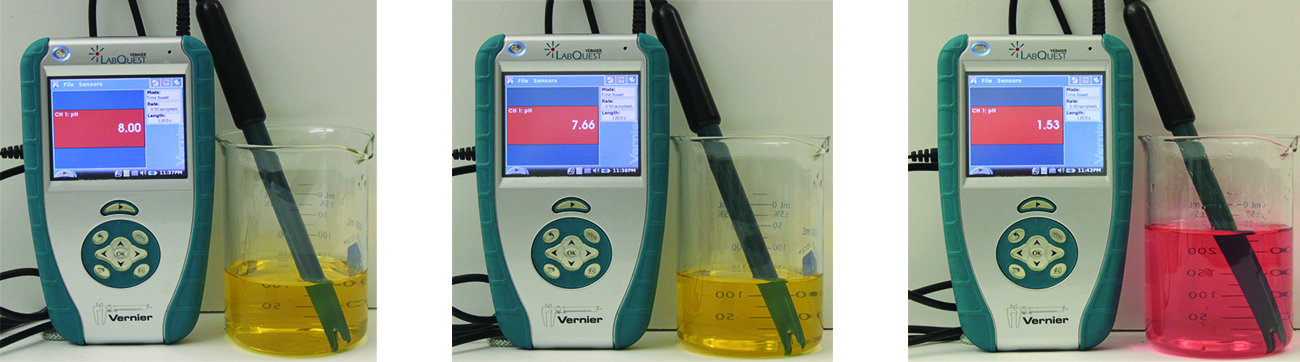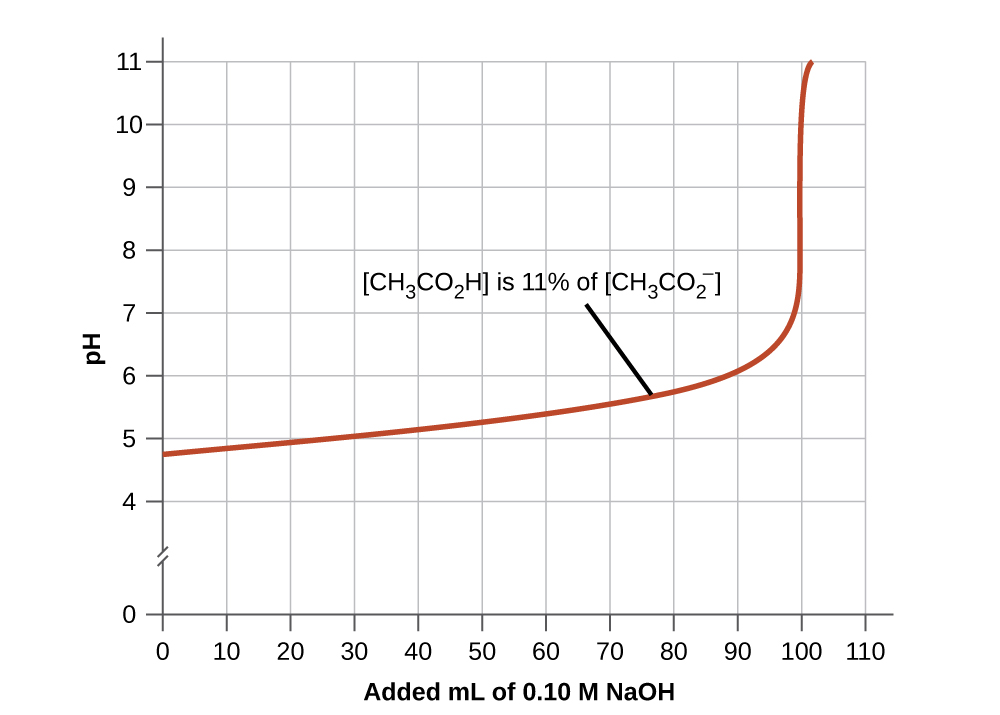# 14.6 Buffers  (Page 3/9)

 Page 3 / 9
$\left(1.0\phantom{\rule{0.2em}{0ex}}×\phantom{\rule{0.2em}{0ex}}{10}^{-4}\right)\phantom{\rule{0.2em}{0ex}}-\phantom{\rule{0.2em}{0ex}}\left(1.8\phantom{\rule{0.2em}{0ex}}×\phantom{\rule{0.2em}{0ex}}{10}^{-6}\right)\phantom{\rule{0.2em}{0ex}}=9.8\phantom{\rule{0.2em}{0ex}}×\phantom{\rule{0.2em}{0ex}}{10}^{-5}\phantom{\rule{0.2em}{0ex}}M$

The concentration of NaOH is:

$\frac{9.8\phantom{\rule{0.2em}{0ex}}×\phantom{\rule{0.2em}{0ex}}{10}^{-5}\phantom{\rule{0.2em}{0ex}}M\phantom{\rule{0.2em}{0ex}}\text{NaOH}}{0.101\phantom{\rule{0.2em}{0ex}}\text{L}}\phantom{\rule{0.2em}{0ex}}=9.7\phantom{\rule{0.2em}{0ex}}×\phantom{\rule{0.2em}{0ex}}{10}^{-4}M$

The pOH of this solution is:

$\text{pOH}=\text{−log}\left[{\text{OH}}^{\text{−}}\right]\phantom{\rule{0.2em}{0ex}}=\text{−log}\left(9.7\phantom{\rule{0.2em}{0ex}}×\phantom{\rule{0.2em}{0ex}}{10}^{-4}\right)\phantom{\rule{0.2em}{0ex}}=3.01$

The pH is:

$\text{pH}=14.00\phantom{\rule{0.2em}{0ex}}-\phantom{\rule{0.2em}{0ex}}\text{pOH}=10.99$

The pH changes from 4.74 to 10.99 in this unbuffered solution. This compares to the change of 4.74 to 4.75 that occurred when the same amount of NaOH was added to the buffered solution described in part (b).

Show that adding 1.0 mL of 0.10 M HCl changes the pH of 100 mL of a 1.8 $×$ 10 −5 M HCl solution from 4.74 to 3.00.

Initial pH of 1.8 $×$ 10 −5 M HCl; pH = −log[H 3 O + ] = −log[1.8 $×$ 10 −5 ] = 4.74
Moles of H 3 O + in 100 mL 1.8 $×$ 10 −5 M HCl; 1.8 $×$ 10 −5 moles/L $×$ 0.100 L = 1.8 $×$ 10 −6
Moles of H 3 O + added by addition of 1.0 mL of 0.10 M HCl: 0.10 moles/L $×$ 0.0010 L = 1.0 $×$ 10 −4 moles; final pH after addition of 1.0 mL of 0.10 M HCl:

$\text{pH}=\text{−log}\left[{\text{H}}_{3}{\text{O}}^{\text{+}}\right]=\text{−log}\left(\phantom{\rule{0.2em}{0ex}}\frac{\text{total moles}\phantom{\rule{0.2em}{0ex}}{\text{H}}_{3}{\text{O}}^{\text{+}}}{\text{total volume}}\right)\phantom{\rule{0.2em}{0ex}}\phantom{\rule{0.2em}{0ex}}=\text{−log}\left(\phantom{\rule{0.2em}{0ex}}\frac{1.0\phantom{\rule{0.2em}{0ex}}×\phantom{\rule{0.2em}{0ex}}{10}^{-4}\phantom{\rule{0.2em}{0ex}}\text{mol}+1.8\phantom{\rule{0.2em}{0ex}}×\phantom{\rule{0.2em}{0ex}}{10}^{-6}\phantom{\rule{0.2em}{0ex}}\text{mol}}{101\phantom{\rule{0.2em}{0ex}}\text{mL}\left(\phantom{\rule{0.2em}{0ex}}\frac{1\phantom{\rule{0.2em}{0ex}}\text{L}}{1000\phantom{\rule{0.2em}{0ex}}\text{mL}}\right)\phantom{\rule{0.2em}{0ex}}}\right)\phantom{\rule{0.2em}{0ex}}\phantom{\rule{0.2em}{0ex}}=3.00$

If we add an acid or a base to a buffer that is a mixture of a weak base and its salt, the calculations of the changes in pH are analogous to those for a buffer mixture of a weak acid and its salt.

## Buffer capacity

Buffer solutions do not have an unlimited capacity to keep the pH relatively constant ( [link] ). If we add so much base to a buffer that the weak acid is exhausted, no more buffering action toward the base is possible. On the other hand, if we add an excess of acid, the weak base would be exhausted, and no more buffering action toward any additional acid would be possible. In fact, we do not even need to exhaust all of the acid or base in a buffer to overwhelm it; its buffering action will diminish rapidly as a given component nears depletion.The indicator color (methyl orange) shows that a small amount of acid added to a buffered solution of pH 8 (beaker on the left) has little affect on the buffered system (middle beaker). However, a large amount of acid exhausts the buffering capacity of the solution and the pH changes dramatically (beaker on the right). (credit: modification of work by Mark Ott)

The buffer capacity    is the amount of acid or base that can be added to a given volume of a buffer solution before the pH changes significantly, usually by one unit. Buffer capacity depends on the amounts of the weak acid and its conjugate base that are in a buffer mixture. For example, 1 L of a solution that is 1.0 M in acetic acid and 1.0 M in sodium acetate has a greater buffer capacity than 1 L of a solution that is 0.10 M in acetic acid and 0.10 M in sodium acetate even though both solutions have the same pH. The first solution has more buffer capacity because it contains more acetic acid and acetate ion.

## Selection of suitable buffer mixtures

There are two useful rules of thumb for selecting buffer mixtures:

1. A good buffer mixture should have about equal concentrations of both of its components. A buffer solution has generally lost its usefulness when one component of the buffer pair is less than about 10% of the other. [link] shows an acetic acid-acetate ion buffer as base is added. The initial pH is 4.74. A change of 1 pH unit occurs when the acetic acid concentration is reduced to 11% of the acetate ion concentration.The graph, an illustration of buffering action, shows change of pH as an increasing amount of a 0.10- M NaOH solution is added to 100 mL of a buffer solution in which, initially, [CH 3 CO 2 H] = 0.10 M and [ CH 3 CO 2 − ] = 0.10 M .
2. Weak acids and their salts are better as buffers for pHs less than 7; weak bases and their salts are better as buffers for pHs greater than 7.

what is chemistry?
forms of biotechnology
What is a mole?
pls give me 3 type of transition metal
Copper, Scandium, Vanadium, Iron, Chromium, Cobalt
Jumaane-Kalif
ion zinc hydrogen
Abdul
that is not true
Jumaane-Kalif
stop posting foolishness
Jumaane-Kalif
how do I name compounds
depends on the compound. as you may know there's transition metal compounds and there's organic compounds and so on and so forth.
Jumaane-Kalif
what is electricity
electricity refers to the flow electrons
John
Hi. please can you tell me more on chemical equation
When 1 or 2 chemicals react, they rearrange their atomic composition forming new compounds. The total mass before and after is the same/ constant. Chemical equations of same reactants react in same ratios e.g. 1 Na ion reacts with 1 water molecule or a multipier like 1 mole of Na ions react with 1
Abdelkarim
Mole of water molecules. In that example we multipied by 6.02*10^23 or avogadro constant (L). Or 2 Na+ ions with two water molecules. The arrow means '' to form '' Some times conditions or reactants are written above the arrow like H+ or enzyme or temper, sometimes physical states are written
Abdelkarim
Beside the chemical Aqueous (aq) which means solute dissolved on water. Solid (s) etc Some chemical equations are written next to it ΔH= # which means enthalpy change which describes if the reaction is endothermic (+) or exothermic (-).
Abdelkarim
Some are reversible and have half double arrow sign.
Abdelkarim
what is the meaning of atoma
what is theory
what is chemical compound
Lorrita
A compound is the result of chemical bonding between 2 or more different elements.
Abdelkarim
Why is an atom electrically neutral?
Clara
the same number of electron , proton present in an atom.thats why it is electrically neutral
debibandita
difference between Amine and amide
what is the difference between alkanal and alkanone
difference between alkanol and alkanal
Michael
whaatt
Saturday
you are not well at all
Ibrahim
is organic compounds used for drying agent
Sulfuric acid is used as a drying agent.
Abdelkarim
what is an atom
An atom is the smallest part of an element, for instance gold atoms are the smallest part of gold that can enter a reaction. An atom must consist protons and electrons of equal number. You can think of those subatomic particles as spheres, but not orbiting randomly they move in specific way in
Abdelkarim
That was partially described mathematically. As a muslim, we believe that god created all matter from nothing. He is the Able, and only who can create matter in the begging in the big bang that was described in the Quran in chapter 17 verse 30, 1400 years ago, you can read it from internet.
Abdelkarim
what is electron affinity
John
do you have a private jet
Ibrahim
what is acid
Proton donor (H+). Like in lemons, oranges and some medicines.
Abdelkarim
what is titration? and how can i get my readings?
what is electrolysis?
what is the formula you use when calculating for gas law?
favor
PV=nrt
Farid
electro-means electricity while lysis-means splitting up so electrolysis simply means splitting up by means of electric current
John
good day. how may I see previous question asked in this chat, please?
Asha
Asha, A person named Favor asked what is meant by the term electrolysis
John

#### Get Jobilize Job Search Mobile App in your pocket Now!ByByBy Stephen VoronBy OpenStaxBy Kevin MoquinBy OpenStaxBy OpenStaxBy Eddie UnverzagtBy John GabrieliBy Brooke DelaneyBy Dionne MahaffeyBy OpenStax